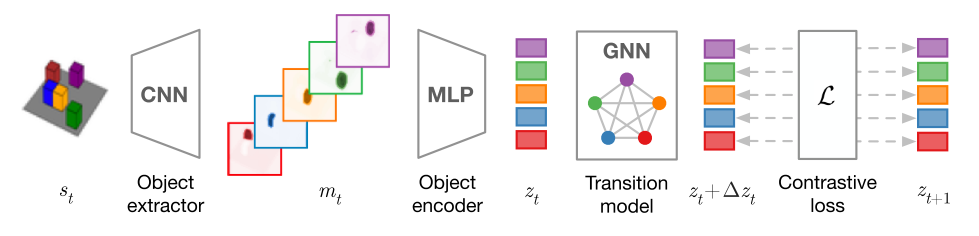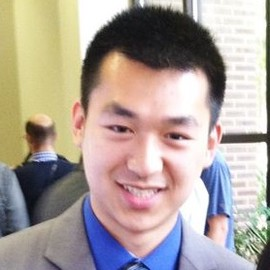# Contrastive Learning of Structured World Models

Kipf et al., 2020Source: Kipf et al., 2020

## Summary

• Contrastively-trained Structured World Models (C-SWMs) learn a world model, in terms of objects, relations, and hierarchies, from raw sensory data
• Utilizes a contrastive approach, which distinguishes real versus fake experiences
• Overcomes limitations of pixel-based methods
• Links: [ website ] [ pdf ]

## Background

• Human compositional reasoning in terms of objects, relations, and actions serves as motivation for developing artificial systems that decompose scenes into similar representations, which greatly facilitate physical dynamics prediction
• Most methods use a generative appraoch, reconstructing visual predictions and optimizing an objective in pixel-space
• This results in ignoring visually small, but significant, features or wasting model capcity on visually rich, but irrelevant, features
• C-SWMs, on the other hand, use a discriminative approach which does not suffer from the same challenges

## Methods

• Consider off-policy setting, using buffer of offline experience
• Object Extractor and Encoder:
• CNN-based object extractor: takes images and produces $K$ features maps, corresponding to the $K$ object slots, which can be interpreted as object masks
• MLP-based object encoder: take a flattened feature map and outputs an abstract state representation, $z^k_t$ – shared across objects
• Relational Transition Model ($T(z_t, a_t)$): graph neural network that models pairwise interactions between object states as well as actions applied to objects
• Multi-object Contrastive Loss: energy-based hinge loss with energy computed by taking the mean energy across the $K$ objects
• Postive samples: $H = \frac{1}{K}\sum^K_{k=1}d(z^k_t+T^k(z_t,a_t), z^k_{t+1})$, where $d(\cdot, \cdot)$ is the squared Euclidean distance
• Negative samples: $\tilde{H} = \frac{1}{K}\sum^K_{k=1}d(\tilde{z}^k_t, z^k_{t+1})$, where $\tilde{z}^k$ is the representation for the corrupted state sampled from buffer
• Final loss: $L = H + max(0, \gamma - \tilde{H})$
• Concatenate two consecutive frames as input for environments with internal dynamics

## Results

• Datasets: random policy used to collect experience
• Novel grid world: 2D and 3D, with multiple interacting objects that can be manipulated
• Atari 2600 games: Atari Pong and Space Invaders
• 3-body physics simulation
• Metrics: based on ranking comparison of predicted state representation to encoded true observation and a set reference states from experience buffer
• Hits at Rank 1 (H@1)
• Mean Reciprocal Rank (MRR)
• Baselines:
• C-SWM ablations: remove latent GNN, latent GNN + factored states, or contrastive loss
• Autoencoder-based World Model: AE or VAE to learn state representations, and MLP to predict action-conditioned next state
• Physics as Inverse Graphics (PAIG): encoder-decoder architecture trained with pixel-based reconstruction, using differentiable physics engine on latent space with explicit position and velocity representations – making it only applicable to 3-body physics environment
• Results summary:
• C-SWMs discover object-specific filters that can be mapped to object positions
• Baselines that use pixel-based reconstruction losses do not generalize as well to unseen scenes
• Removing GNN reduces performance on grid world datasets for longer time horizons, but no for single step
• Removing factored states or contrastive loss reduces performance drastically
• Performance is basically at ceiling for non-Atari datsets, even for non-ablation baselines
• For Atari datasets, C-SWM outperforms AE-based World Model for 1 step predictions, but is reliant on tuning of $K$
• For longer time horizons C-SWM is better, but that’s expected due to the GNN vs. MLP

## Conclusion

• Object extractor relies on distinct visual features to disambiguate objects
• No method to ensure there isn’t redundancy between the $K$ object feature maps
• The contrastive loss as an alternative to pixel-based losses is promising